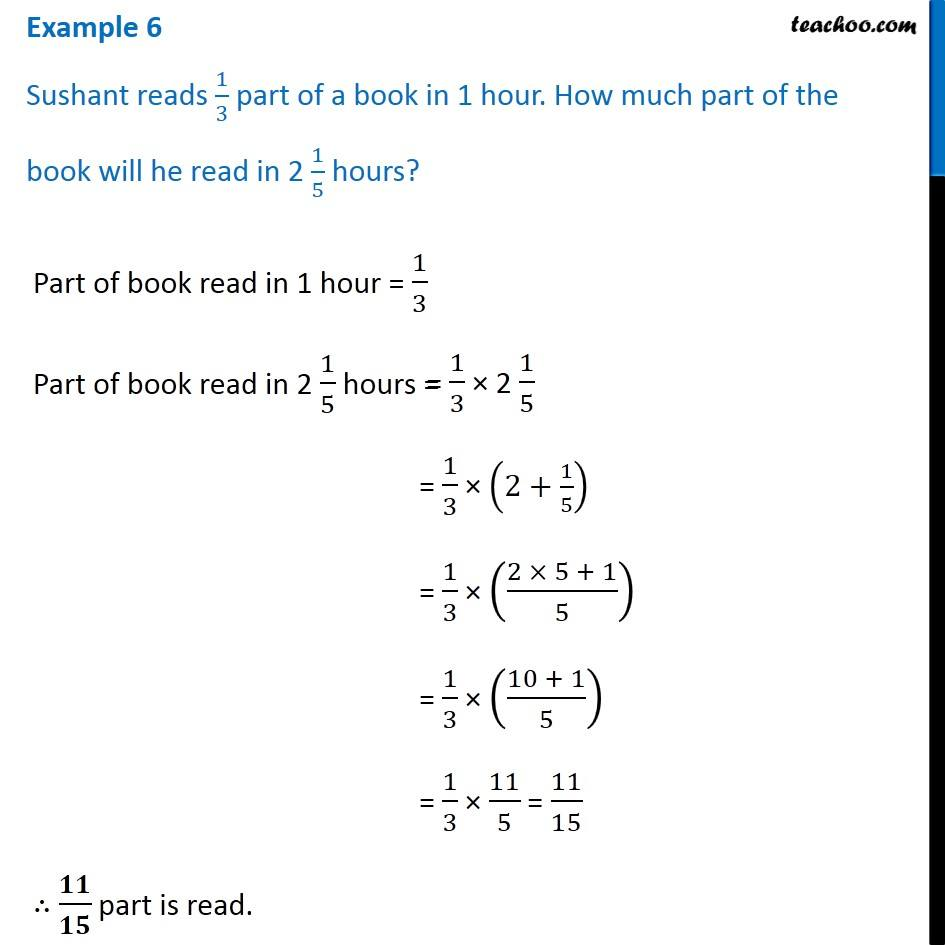1. Chapter 2 Class 7 Fractions and Decimals
2. Serial order wise
3. Examples

Transcript

Example 6 Sushant reads 1/3 part of a book in 1 hour. How much part of the book will he read in 2 1/5 hours?Part of book read in 1 hour = 1/3 Part of book read in 2 1/5 hours = = 1/3 × (2+1/5) = 1/3 × ((2 × 5 + 1)/5) = 1/3 × ((10 + 1)/5) = 1/3 × 11/5 = 11/15 ∴ 𝟏𝟏/𝟏𝟓 part is read.

Examples